OS

Machine learning
Explainability

# How LIME works | Understanding in 5 steps

## Leveraging how LIME works to build trustworthy ML

Share

Being able to produce explainable predictions lies at the center of trustworthy machine learning (ML). LIME, which is short for Local Interpretable Model-agnostic Explanations, is a technique that can be used to explain the predictions made by any black-box classifier.

In this post, we start by discussing why explainability matters in ML. Then, we understand how LIME works by going through the five steps followed by the algorithm to explain a model prediction. Finally, we illustrate a simple use of LIME in churn prediction and give pointers to references for further exploration, if this is a topic you are interested in.

Learn the secrets of performant and explainable ML

Be the first to know by subscribing to the blog. You will be notified whenever there is a new post.

## Why explainability is useful

There is often a trade-off between interpretability and predictive performance when choosing between different ML models for a task.

The consequences of such a trade-off appear in the types of ML approaches chosen by the industry. For example, in highly regulated fields where there must always be a way to justify the model’s predictions, interpretable models — such as shallow decision trees or linear models with sparse features — are often chosen over more powerful alternatives.

LIME is a technique that can be used with any black-box classifier. Its usefulness is based on the fact that instead of having to work only with intrinsically interpretable models and their limited capabilities, one can use any classifier and then generate explanations for individual predictions afterward, thus benefiting from the best of both worlds.

## How LIME works

To produce the explanations, LIME feeds the black-box model with small variations of the original data sample and probes how the model’s predictions change. From these variations, it learns an interpretable model which approximates the black-box classifier in the vicinity of the original data sample. Locally, the interpretable model provides a faithful approximation of the black-box model, even though it is likely not a globally good approximator.

Don’t worry if you didn’t understand everything by just reading the previous description. Let's go through the five steps followed by LIME to generate an explanation.

### Step 1: Choosing the sample to be explained

Consider the binary classification task from two features. Furthermore, let’s say we have a black-box classifier illustrated below, where class 0 is in red and class 1 is in blue.

The first step in using LIME is choosing the data sample to be explained. For example, this could be a point from your validation set that your model made a mistake or, really, any sample that you are interested in investigating further.

In this case, we are interested in understanding why the black-box classifier predicted that a specific sample, x', belongs to class 0.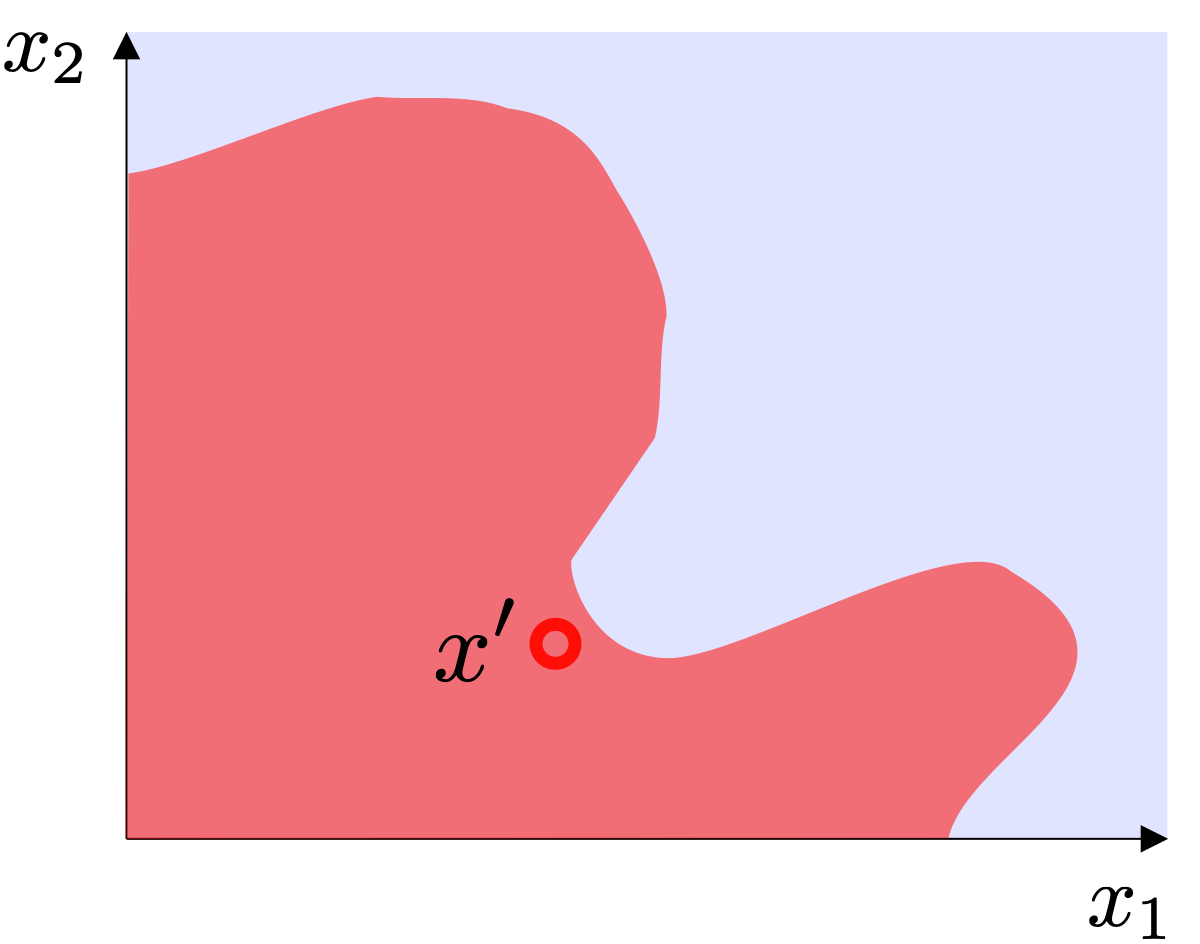### Step 2: Perturbing the sample

Now, the actual LIME algorithm kicks in. It starts by randomly perturbing the data sample x', which results in multiple new samples, depicted as the black dots in the image below.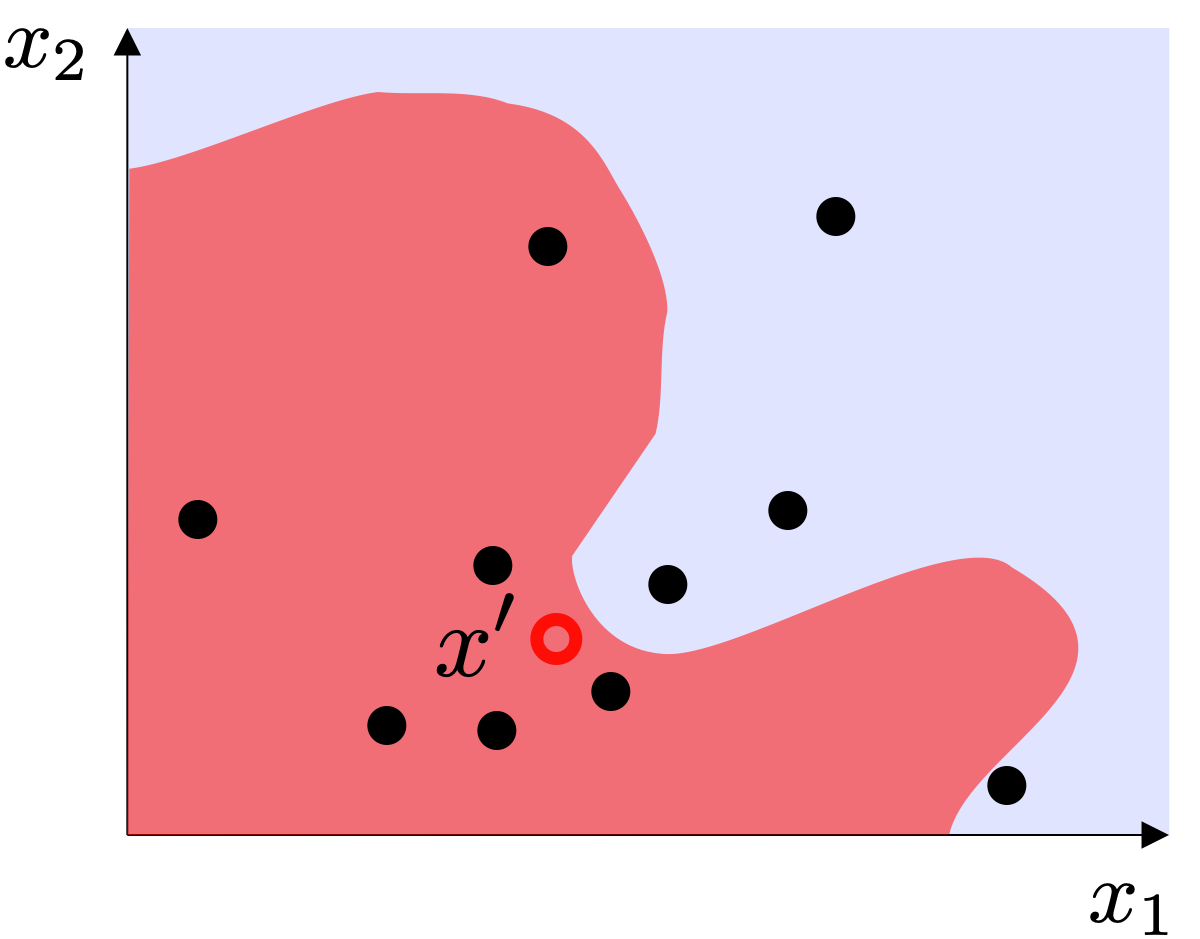### Step 3: Labelling the perturbed samples with the black-box model

Then, these perturbed samples are given to the black-box model, to see how it would predict them. These labeled samples will serve as a dataset to train an interpretable model.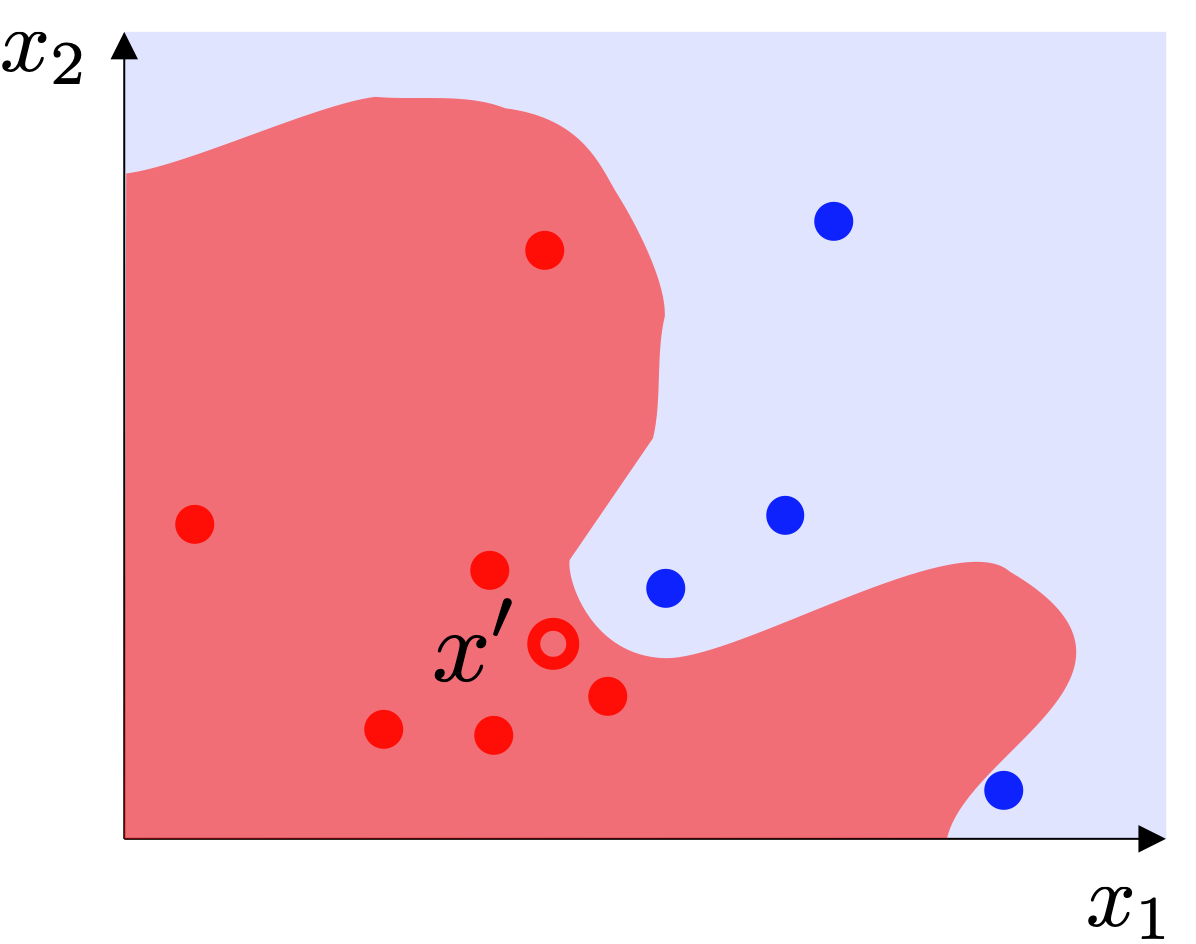### Step 4: Weighting

There is an additional catch. Since one of the premises that LIME builds upon is that the interpretable model can be a good local approximation, we are mostly interested in the perturbed samples that are close to x'. Therefore, to learn the interpretable model, LIME weights the samples according to their proximity to x', so samples close to x' are given a large weight, and samples far from x' are given a small weight.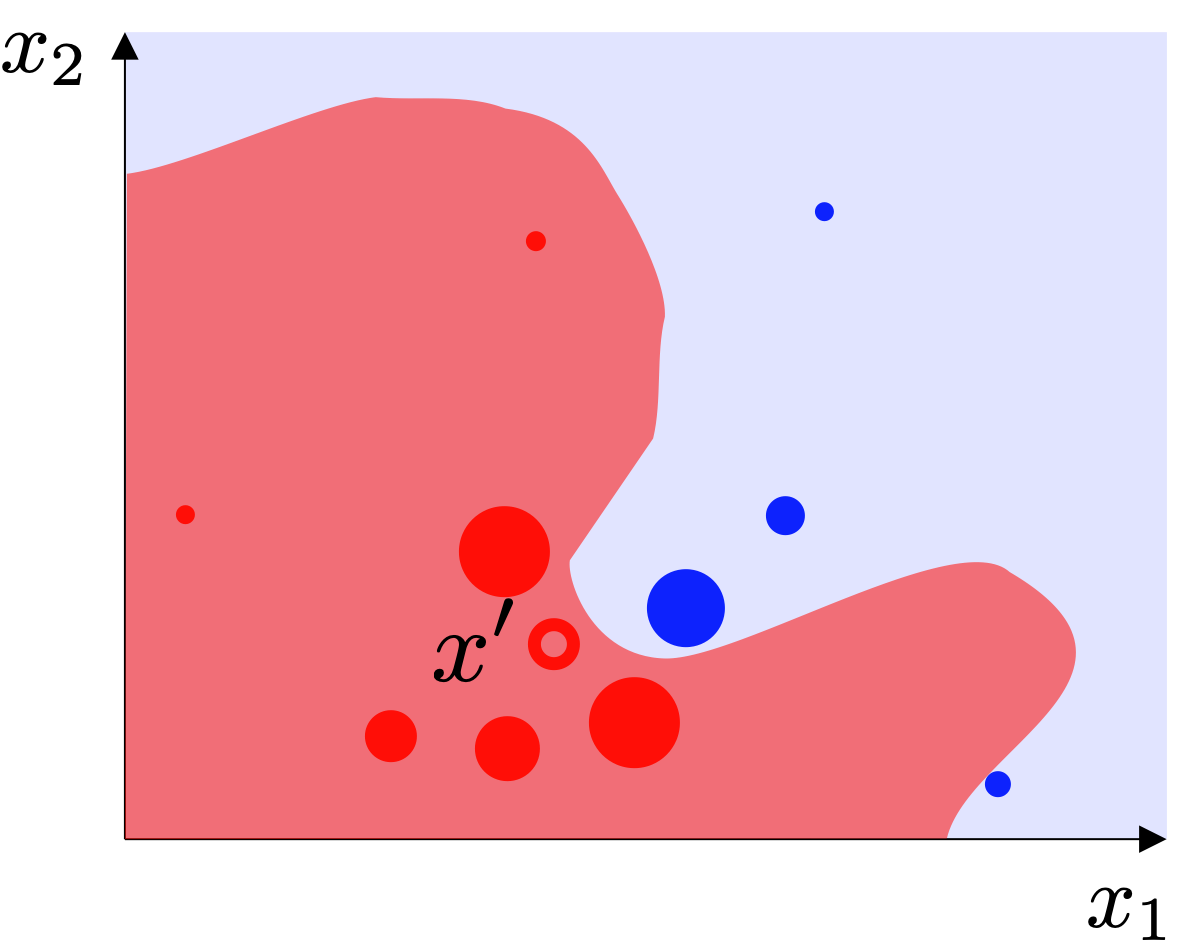### Step 5: Learning the interpretable model

Finally, LIME is ready to learn the interpretable model!

The default interpretable model used by LIME is a linear model. It is learned from the weighted samples and provides explanations for a particular prediction, in this case, x'. Even though the black-box model might be nonlinear, we expect that close to x', the decision boundary can be reasonably approximated by a line.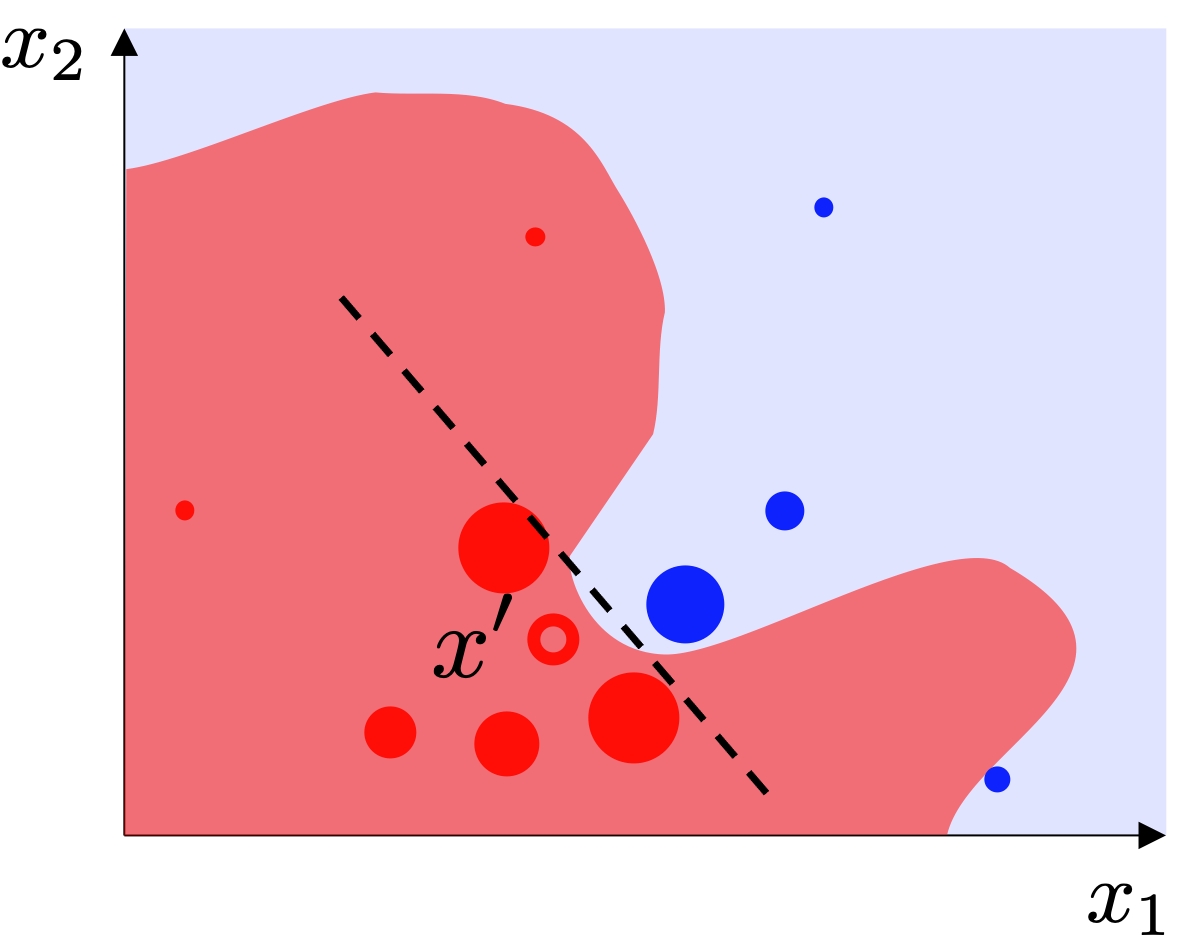A linear model with sparse features, such as the one learned by LIME, is intrinsically interpretable. The model’s weights can be inspected and seen as proxies for feature importance. Therefore, what LIME returns as the feature scores is actually the linear model’s weights.

For the sake of simplicity, in this post, we focused on giving a high-level overview of how LIME works for tabular data with only two features, avoiding the mathematical details from the problem formulation. The generalization from two features to many is direct, we just wouldn’t be able to draw nice figures.

Moreover, LIME works not only for tabular data but also for text and image data as well. There are a few modifications required so that the data representation over which the explanations are provided remains easily interpretable to humans. All of these modifications are discussed in more detail in the Interpretable Data Representations section from the original paper and the interested reader is encouraged to check it out.

## Churn prediction with LIME

LIME is a groundbreaking technique that changed the landscape of ML interpretability.

Let’s go through a simple example that illustrates the usefulness of explainability in a real-world situation. The code and data for this example are available on GitHub.

Imagine you have trained a model that predicts whether a user is likely to churn from demographic and usage data. The resulting model often mistakenly predicts a user is going to churn when, in fact, it doesn't.

To diagnose the problem, you want to understand a few of your model’s mispredictions.

Let's inspect the explainability scores generated by LIME for a row in the validation set.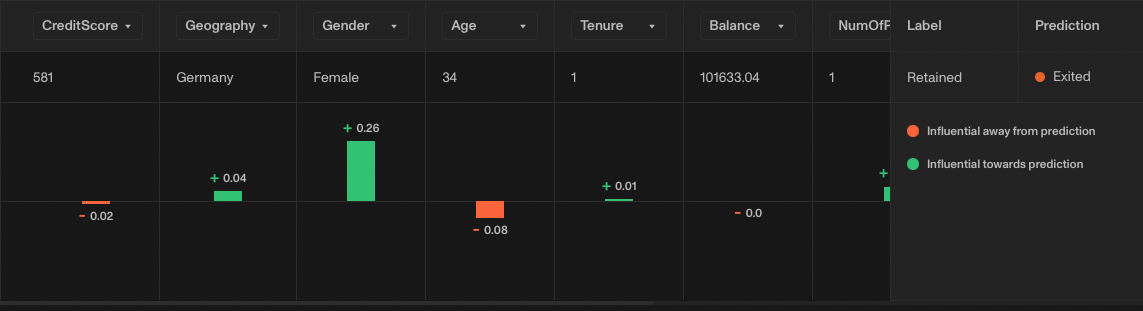(Screenshot from Openlayer.)

Note how for this example, the feature that contributed the most to the prediction (shown in green) is the Gender, which in this case is equal to Female.

Inspecting more rows where the model makes exactly the same mistake, we notice that when Gender is not equal to Female, the model doesn't attribute a lot of importance to the Gender. This suggests that the model might have picked up a bias from the training set.

Actionable insights arise naturally once we leverage the power of explanations. Moreover, explanations build trust that your model is behaving as expected and not simply over-indexing to certain features, for instance.